# 802.11ax PHY-Focused System-Level Simulation

This example shows how to perform a PHY-focused system-level simulation for IEEE® 802.11ax™. Part (A) validates the simulation scenario, radio characteristics, and large-scale fading model by comparing against published calibration results. Part (B) estimates the packet error rate of the 802.11ax network by simulating individual links between active nodes under a basic clear channel assessment scheme.

### Introduction

In this example the performance of an 802.11ax [ 1 ] network in a residential apartment block is evaluated using a PHY-focused system-level simulation.

The residential apartment block simulation scenario is specified in [ 2 ]. This consists of a building with five floors, and twenty 10m x 10m x 3m apartments per floor. Each apartment has an access point (AP) and one or more stations (STAs) placed in random xy-locations, a process referred to as 'dropping' nodes. This creates a basic service set (BSS) per apartment which is randomly assigned one of three channels. The simulation scenario specifies a large-scale path loss model based on the distance between nodes, and the number of walls and floors traversed.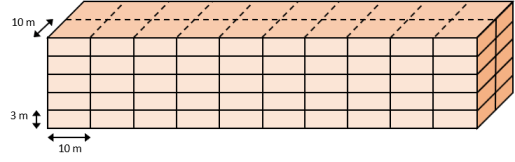The TGax evaluation methodology [ 3 ] for 'PHY System Simulation' is followed for this example:

1. APs and STAs are randomly 'dropped' within the scenario.

2. For each pair of nodes, the large-scale path loss is calculated.

3. One or more 'transmission events' is performed. Each transmission event consists of selecting active APs and STAs based on channel access rules and determining the performance of each link.

This example consists of two parts:

In part (A), the 'calibration' stage, the signal-to-interference-plus-noise ratio (SINR) is calculated for multiple 'drops', assuming downlink interfering transmissions. SINR captures long-term radio characteristics. The cumulative distribution function (CDF) of the SINR is compared with published results from the TGax Task Group [ 4 ].

In part (B), the 'PHY system-level simulation' stage, for each transmission event the PHY layer is modeled for individual links. A basic clear channel assessment (CCA) scheme is used to control which APs are active. Waveforms for the signal of interest and interference, impaired by fading channel models, are generated and combined. The resultant packets are processed by a receiver to recover the packet of interest. The average packet error rate for the network is calculated.

The two parts of this example can be disabled using the parameters `calibrate` and `systemLevelSimulation`. A figure displaying the simulation scenarios, nodes, active links, and interference is displayed when `showScenarioPlot` is true.

```calibrate = true; % To execute Part A calibration test systemLevelSimulation = true; % To execute Part B system-level simulation showScenarioPlot = true; % To show dynamic simulation plotting updates ```

### Simulation Parameters

The major simulation parameters are defined as either belonging to Physical Layer (PHY), Medium Access Control Layer (MAC), scenario, or simulation. In this example the PHY and MAC parameters are assumed to be the same for all nodes.

```PHYParameters = struct; PHYParameters.TxPower = 20; % Transmitter power in dBm PHYParameters.TxGain = 0; % Transmitter antenna gain in dBi PHYParameters.RxGain = -2; % Receiver antenna gain in dBi PHYParameters.NoiseFigure = 7; % Receiver noise figure in dB PHYParameters.NumTxAntennas = 1; % Number of transmitter antennas PHYParameters.NumRxAntennas = 1; % Number of receiver antennas PHYParameters.ChannelBandwidth = 'CBW80'; % Bandwidth of system PHYParameters.TransmitterFrequency = 5e9; % Transmitter frequency in Hz MACParameters = struct; MACParameters.NumChannels = 3; % Number of non-overlapping channels MACParameters.CCALevel = -70; % Transmission threshold in CCA algorithm (dBm) ```

The scenario parameters define the size and layout of the residential building as per .

```% Number of Rooms in [x,y,z] directions ScenarioParameters = struct; ScenarioParameters.BuildingLayout = [10 2 5]; % Size of each room in meters [x,y,z] ScenarioParameters.RoomSize = [10 10 3]; % Number of receivers per room. Note that only one receiver (STA) can be % active at any given time. ScenarioParameters.NumRxPerRoom = 1; ```

The `NumDrops` and `NumTxEventsPerDrop` parameters control the length of the simulation. For this example, these parameters are configured for a short simulation, but for meaningful results these should be increased.

A 'drop' randomly places transmitters and receivers within the scenario and selects the channel for a BSS. A 'transmission' event randomly selects transmitters and receivers for transmissions according to basic channel access rules.

```SimParameters = struct; SimParameters.NumDrops = 3; SimParameters.NumTxEventsPerDrop = 2; ```

### Generate Transmitter Sites

Before the main body of the simulation, the transmitter site objects `txsite` are generated and assigned room names of the form 'Room#' for ease of reference. One transmitter (AP) per room is assumed. Each transmitter is assumed to be isotropic.

```% Total number of transmitters, assuming one transmitter (tx) per room numTx = prod(ScenarioParameters.BuildingLayout); % Create transmitter sites with and isotropic antenna element roomNames = strings(1,numTx); for siteInd = 1:numTx roomNames(siteInd) = "Room " + siteInd; end txs = txsite('cartesian','Name',roomNames,... 'TransmitterFrequency',PHYParameters.TransmitterFrequency, ... 'TransmitterPower',10.^((PHYParameters.TxPower+PHYParameters.TxGain-30)/10),... 'Antenna','isotropic'); ```

### Generate Receiver Sites

The receive site objects `rxsite` are generated and assigned names of the form 'Room#-STA#' for ease of reference. The Scenario parameter `NumRxPerRoom` is used to define how many receivers (STAs) are present in each room. Each receiver is assumed to be isotropic.

```% Total number of receivers, assuming one transmitter (tx) per room numRx = numTx*ScenarioParameters.NumRxPerRoom; % Create receiver sites roomNames = strings(1,numRx); for siteInd = 1:numRx roomNames(siteInd) = "Room " + (mod(siteInd-1,numTx)+1) + "-" + ceil(siteInd/numTx); end rxs = rxsite('cartesian','Name',roomNames,'Antenna','isotropic'); % Receiver noise power in dBm T = 290; % Temperature (Kelvin) k = physconst('Boltzmann'); % Boltzmann constant % Sample rate (Hz) fs = wlanSampleRate(wlanHESUConfig('ChannelBandwidth',PHYParameters.ChannelBandwidth)); rxNoisePower = 10*log10(k*T*fs)+30+PHYParameters.NoiseFigure; ```

### Part A - Align Long-Term Radio Characteristics

In this section, the simulation scenario, radio characteristics, and large-scale fading model are verified by performing the TGax Evaluation Methodology Box 1 Test 2 Downlink Only calibration test [ 3 ]. This test calculates the SINR at all receivers (STAs) assuming all transmitters (APs) are active. Multiple drops of transmitters and receivers are performed as part of the simulation. One active receiver is selected per drop.

The SINR for each receiver is calculated and aggregated over all drops simulated to generate a CDF curve. This curve is compared with the calibration results provided in [ 4 ].

A plot showing the node positions, active links, and interfering links is generated per drop. Individual channels can be hidden and shown in the plot by clicking the corresponding legend entry.

```seed = rng(6); % Seed random number generator and store state if showScenarioPlot hGrid = tgaxBuildResidentialGrid(ScenarioParameters.RoomSize,ScenarioParameters.BuildingLayout, ... numTx,numRx,MACParameters.NumChannels); end if calibrate fprintf('Running calibration ...\n'); % Pre-allocate output output = struct; output.sinr = zeros(SimParameters.NumDrops,numTx); % for storing SINR values for drop = 1:SimParameters.NumDrops % Drop receivers in each room [association,txChannels,rxChannels,txPositions,rxPositions] = tgaxDropNodes( ... txs,rxs,ScenarioParameters,MACParameters.NumChannels); % All transmitters active activeTx = true(numTx,1); % Only pick one receiver per Room rxAlloc = randi([1 ScenarioParameters.NumRxPerRoom],numTx,1); activeRx = reshape(rxAlloc==1:ScenarioParameters.NumRxPerRoom,[],1); % Generate propagation model propModel = TGaxResidential('roomSize',ScenarioParameters.RoomSize); % Get the index of the transmitter for each receiver tnum = repmat((1:numTx),1,numRx/numTx); % SINR calculation - loop over each non-overlapping channel activeChannels = unique(txChannels); for k = 1:numel(activeChannels) % Use kth non-overlapping channel tind = txChannels == activeChannels(k); rind = false(size(activeRx)); rind(activeRx) = rxChannels(activeRx) == activeChannels(k); % Get the index of the transmitter of interest for each active receiver tsigind = tnum(rind); % Calculate SNR output.sinr(drop,tind) = sinr(rxs(rind),txs(tind),... 'ReceiverGain',PHYParameters.RxGain,... 'ReceiverNoisePower',rxNoisePower,... 'PropagationModel',propModel,... 'SignalSource',txs(tsigind)); end % Plot nodes and links if showScenarioPlot % update plot data mask = txChannels==rxChannels'; tgaxUpdatePlot(hGrid,txPositions,rxPositions,activeTx,activeRx,mask,txChannels,rxChannels, ... sprintf('Box 1 Test 2 "downlink only" calibration, drop #%d/%d',drop,SimParameters.NumDrops)); end end % Plot the CDF of SINR and compare with calibration curves tgaxCalibrationCDF(output.sinr,'SS1Box1Test2','Long-term Radio Characteristics'); fprintf('Calibration complete \n') end ```
```Running calibration ... Calibration complete ```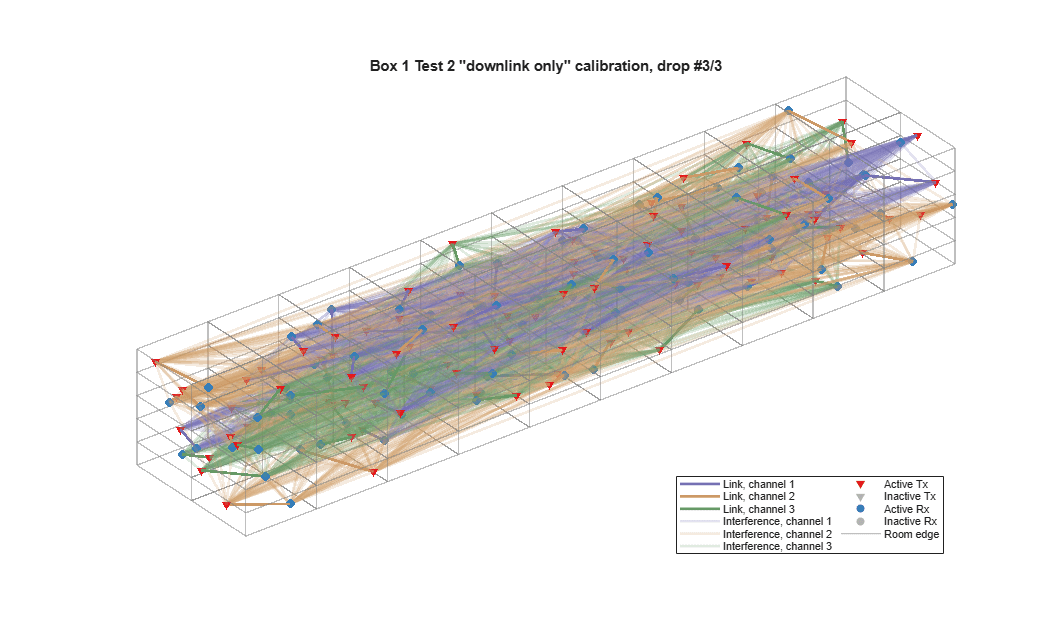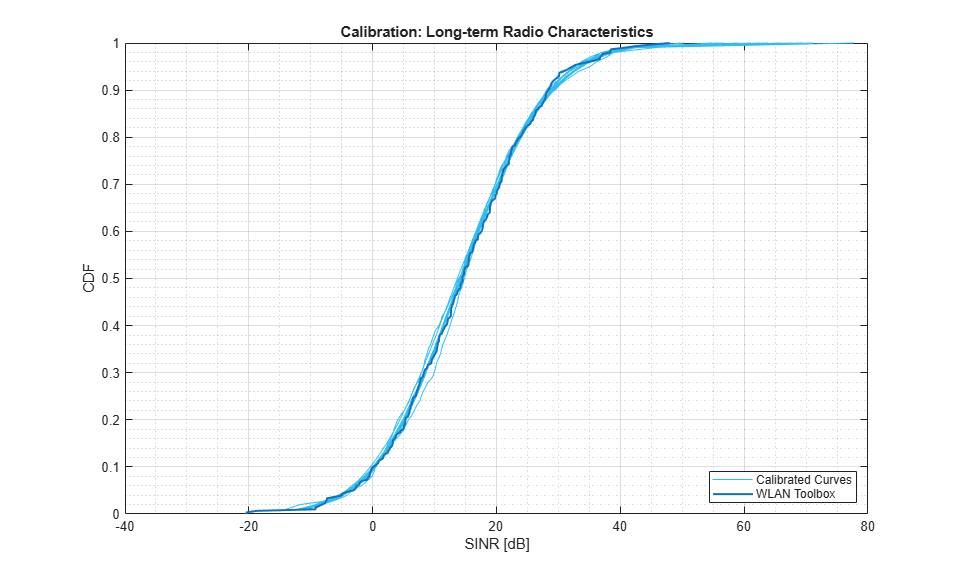This example simulates a small number of drops. Therefore, for a more meaningful comparison the number of drops simulated should be increased. The calibration result for 100 drops is shown below: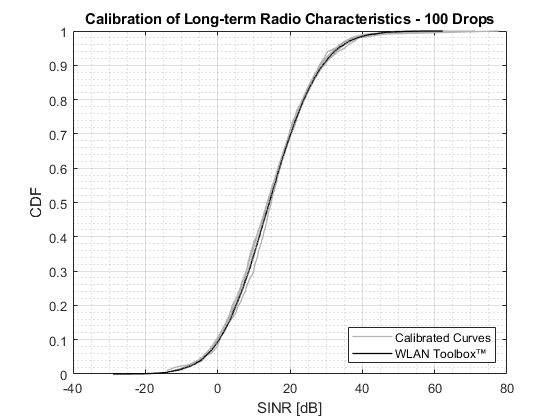### Part B - PHY-focused System-Level Simulation

In this section, the scenario and path-loss model calibrated in part A are used to perform a PHY-focused system-level simulation and determine the packet error rate for the network. This simulation is described as PHY-focused as the PHY is not abstracted and the MAC is simplified. Each active link is modeled using baseband transmitter and receiver processing. A very simple MAC assumes at each transmission event all transmitters (APs) wish to transmit, and one receiver (STA) per BSS is the recipient. A simple CCA algorithm is used to control channel access between transmitters as specified in Figure 4 of [ 3 ]. The CCA algorithm enables random transmitters if the signal power received from transmitters that have already been activated does not exceed the CCA threshold, `MACParameters.CCAThreshold`.

The received signal power from all possible interfering transmitters is calculated at each active receiver. If the received power from an interfering transmitter is above the noise floor of the receiver, then the link is modeled with full baseband transmitter and receiver processing. For each modeled link an HE single-user packet is generated and passed through a TGax Model-D NLOS stationary indoor channel model. At the receiver, the waveforms from the transmitter of interest and all interfering transmitters are scaled by the expected path-loss and combined to create a waveform containing the signal of interest plus interference. All waveforms are time aligned. The receiver performs synchronization, demodulation, and decoding to attempt to recover the payload. The decoded payload is compared to the PSDU transmitted in the BSS to determine if the packet has been recovered successfully.

In this example the transmission and channel parameters are assumed to be the same for all nodes. The transmission configuration for all packets is one space-time stream, no space-time block coding and 16-QAM rate-1/2 (MCS 3).

```if systemLevelSimulation % Pre-allocate outputs output.numPkts = zeros(numRx,1); output.numPktErrors = zeros(numRx,1); output.sinrMeas = nan(numRx,SimParameters.NumTxEventsPerDrop,SimParameters.NumDrops); output.sinrEst = nan(numRx,SimParameters.NumTxEventsPerDrop,SimParameters.NumDrops); output.pktErrorRate = 0; % For each possible transmitter create a waveform configuration. In this % example the link and radio parameters are the same for all nodes. cfgHEBase = wlanHESUConfig; cfgHEBase.ChannelBandwidth = PHYParameters.ChannelBandwidth; % Channel bandwidth cfgHEBase.NumTransmitAntennas = PHYParameters.NumTxAntennas; % Number of transmit antennas cfgHEBase.SpatialMapping = 'Fourier'; % Spatial mapping matrix cfgHEBase.NumSpaceTimeStreams = 1; % Number of space-time streams cfgHEBase.GuardInterval = 0.8; % Guard interval duration cfgHEBase.HELTFType = 4; % HE-LTF compression mode cfgHEBase.APEPLength = 1e3; % Payload length in bytes cfgHEBase.ChannelCoding = 'LDPC'; % Channel coding cfgHEBase.MCS = 3; % Modulation and coding scheme cfgHE = cell(numTx,1); for txidx = 1:numTx cfgHE{txidx} = cfgHEBase; end fprintf('Running System Level Simulation ...\n') for drop = 1:SimParameters.NumDrops fprintf(' Running drop #%d/%d ...\n',drop,SimParameters.NumDrops); % Drop receivers in each room [association,txChannels,rxChannels,txPositions,rxPositions] = tgaxDropNodes( ... txs,rxs,ScenarioParameters,MACParameters.NumChannels); % Generate propagation model propModel = TGaxResidential('roomSize',ScenarioParameters.RoomSize); % Calculate signal strength for all links signalStrength = sigstrength(rxs,txs,propModel,'Type','power',... 'ReceiverGain',PHYParameters.RxGain); % all signal strengths in dBm % Threshold signals below noise level to reduce simulation time signalStrength(signalStrength < rxNoisePower) = -Inf; % Threshold signals that are not on same non-overlapping channel signalStrength(~(txChannels == rxChannels')) = -Inf; % Mask the transmitter-receiver links that are non-negligible to % simulate and get the linear indices nonnegligibleMask = signalStrength > -Inf; % Reset the non-negligible channels to create a new realization for the % current drop nonnegligibleIdx = find(nonnegligibleMask)'; % For each possible active link in a drop create a channel % configuration. In this example the link and radio parameters are the % same for all nodes. tgaxChan = cell(numel(nonnegligibleIdx),1); for i = 1:numel(nonnegligibleIdx) % Index of transmitter for a given link txIdx = mod(nonnegligibleIdx(i)-1,numTx)+1; % Channel configuration. The channel realization for each link is % different as the global random stream is used. tgaxChanBase = wlanTGaxChannel; tgaxChanBase.DelayProfile = 'Model-D'; tgaxChanBase.NumTransmitAntennas = cfgHE{txIdx}.NumTransmitAntennas; tgaxChanBase.NumReceiveAntennas = PHYParameters.NumRxAntennas; tgaxChanBase.TransmitReceiveDistance = 10; % Distance in meters for NLOS tgaxChanBase.ChannelBandwidth = cfgHE{txIdx}.ChannelBandwidth; tgaxChanBase.LargeScaleFadingEffect = 'None'; tgaxChanBase.EnvironmentalSpeed = 0; % m/s, stationary tgaxChanBase.SampleRate = fs; tgaxChanBase.NormalizeChannelOutputs = false; % Store in cell array and reset the channel to generate a new % response tgaxChan{i} = tgaxChanBase; reset(tgaxChan{i}); end for txevent = 1:SimParameters.NumTxEventsPerDrop fprintf(' Running transmission event #%d/%d ...\n',txevent,SimParameters.NumTxEventsPerDrop); % Determine active transmitters and receivers with Clear Channel Assessment [activeTx,activeRx] = tgaxCCA(signalStrength,MACParameters.CCALevel); % Plot scenario and links if showScenarioPlot tgaxUpdatePlot(hGrid,txPositions,rxPositions,activeTx,activeRx,nonnegligibleMask,txChannels,rxChannels, ... sprintf('PHY System-Level Simulation, Drop #%d/%d, Transmission Event #%d/%d', ... drop,SimParameters.NumDrops,txevent,SimParameters.NumTxEventsPerDrop)); end % Extract elements for active links using activeTx and activeRx cfgHEActive = cfgHE(activeTx); associationActive = association(activeTx,activeRx); nonnegligibleMaskActive = nonnegligibleMask(activeTx,activeRx); signalStrengthActive = signalStrength(activeTx,activeRx); % Create array containing active channels tgaxChanActive = cell(size(associationActive)); matchIdx = nonnegligibleIdx==find(activeTx&activeRx'); tgaxChanActive(nonnegligibleMaskActive) = tgaxChan(any(matchIdx,1)); % Generate a waveform for each non-negligible active link and % combine waveforms for each receiver [rxWavs,txPSDUActive,signalPower,interfPower] = tgaxGenerateRxWaveforms( ... cfgHEActive,tgaxChanActive,nonnegligibleMaskActive,signalStrengthActive,associationActive); % Run PHY link simulation for each link and determine if the packet % has been decoded successfully. The estimated interference power % is passed to the receiver in the place of an interference power % measurement algorithm. numActiveRxs = sum(activeRx); pktError = false(numActiveRxs,1); sinrMeas = nan(numActiveRxs,1); for rxIdx = 1:numActiveRxs [pktError(rxIdx),sinrMeas(rxIdx)] = tgaxModelPHYLink( ... rxWavs{rxIdx},cfgHEActive{rxIdx},rxNoisePower,interfPower(rxIdx),txPSDUActive{rxIdx}); end % Store output for active receivers output.numPktErrors(activeRx) = output.numPktErrors(activeRx)+pktError; output.numPkts(activeRx) = output.numPkts(activeRx) + 1; output.sinrMeas(activeRx,txevent,drop) = sinrMeas; % Calculate the expected SINR at each receiver sinrEst = 10*log10(signalPower./(interfPower+10^((rxNoisePower-30)/10))); output.sinrEst(activeRx,txevent,drop) = sinrEst; end end % Calculate average packet error rate output.pktErrorRate = sum(output.numPktErrors)/sum(output.numPkts); disp('Simulation complete') disp(['Average packet error rate for transmitters: ' num2str(output.pktErrorRate)]); end rng(seed); % Restore random state ```
```Running System Level Simulation ... Running drop #1/3 ... Running transmission event #1/2 ... Running transmission event #2/2 ... Running drop #2/3 ... Running transmission event #1/2 ... Running transmission event #2/2 ... Running drop #3/3 ... Running transmission event #1/2 ... Running transmission event #2/2 ... Simulation complete Average packet error rate for transmitters: 0.054152 ```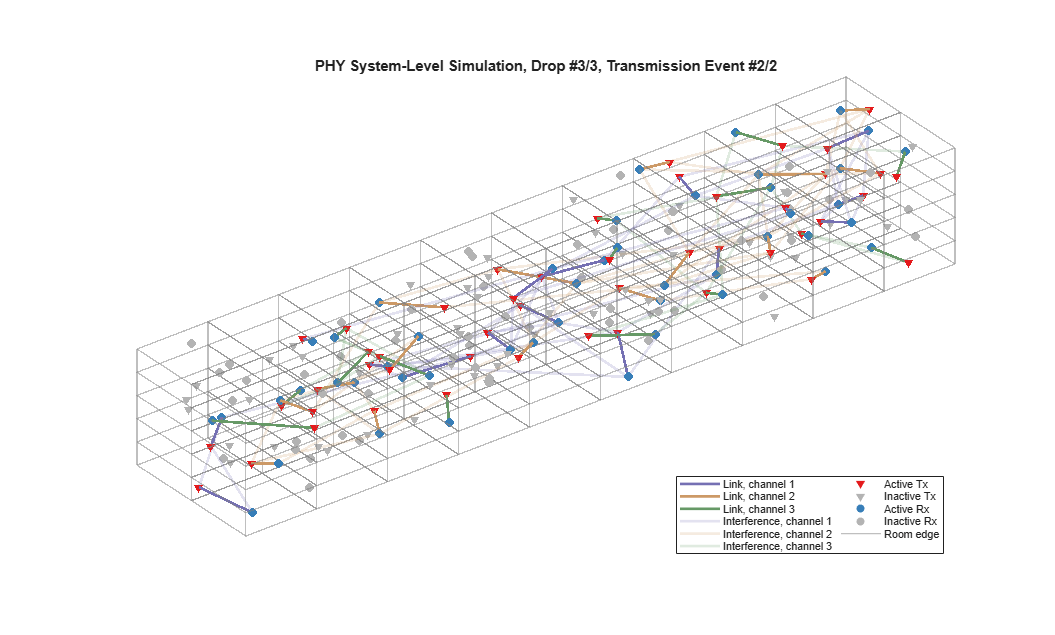### Further Exploration

The PHY-focused system-level simulation demonstrated in this example can be used to explore the impact of PHY-level parameters on system performance. For example, the plot below shows the network average packet error rate for different values of CCA threshold for 50 drops and 2 transmission events per drop.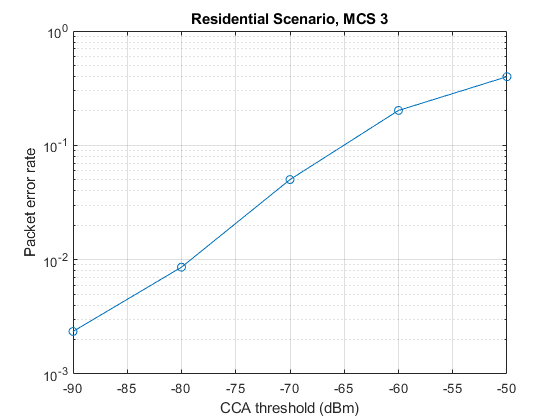### Appendix

This example uses the following helper functions:

### Selected Bibliography

1. IEEE Std 802.11ax™-2021. IEEE Standard for Information Technology - Telecommunications and Information Exchange between Systems - Local and Metropolitan Area Networks - Specific Requirements - Part 11: Wireless LAN Medium Access Control (MAC) and Physical Layer (PHY) Specifications - Amendment 1: Enhancements for High-Efficiency WLAN.

2. IEEE 802.11-14/0980r16 - TGax Simulation Scenarios.

3. IEEE 802.11-14/0571r12 - 11ax Evaluation Methodology.

4. IEEE 802.11-14/0800r30 - Box 1 and Box 2 Calibration Results.